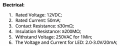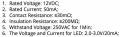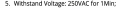# LEDs and potentiometer

#### jono123

Joined Nov 18, 2021
1
Hello,

I wanted to ask a pretty simple question about the usage of a potentiometer with four LEDs in a series circuit.

I've drawn up a simple series circuit with 4 identical LEDs, 1x 10k Ohm potentiometer and a lab PSU set to 12V. Circuit below with info about the LED.As I understand, the circuit shall work but another resistor is ideal so that I do not blow the LEDs when the pot is set to the minimum, i.e. 0 Ohm...

Question: For four LEDs with the electrical info stated, what would be the recommended absolute minimum additional resistor value to add into this circuit so I do not blow the LEDs when the 10k pot is at the minimum position?

Question 2: If I increased the number of LEDs to 73 (total), what would be the recommended minimum resistor value to add into the circuit, like question 1? Can you show mathematically how to calculate?Appreciate your help for a pretty simple question I am sure!!

#### djsfantasi

Joined Apr 11, 2010
8,256
First, since these are 12V LEDs, putting four in series with a 12V supply won’t work. The series circuit would require 48V (4x12) to operate. Voltage requirements in series add; in parallel, each will get the supply voltage. I started to calculate the resistor value when I noticed this problem.

Second, why do you want a potentiometer? If it’s to sum the LEDs, again it won’t work. LEDs are current driven, not voltage driven. To dim LEDs requires a more complicated circuit in order to drive them with PWM (Pulse Width Modulation).

#### Audioguru again

Joined Oct 21, 2019
4,536
Each LED is 2V to 3V, not 12V each!
Four 2V LEDs in series use 8V then the resistor in series with the pot must be (12V - 8V)/20mA= 200 ohms. If the LEDs are 3V each then the current in the 200 ohms resistor might be very low resulting in dim LEDs.

Instead use two parallel strings of two LEDs in series. Each string has a 390 ohms resistor and use a 5k pot. Then there will not be dim LEDs if they are each 3V. Then try it with the n10k pot.

#### dl324

Joined Mar 30, 2015
14,318
Welcome to AAC!

What do the electrical specs represent? What is rated for 12VCD? LEDs don't have contact resistance.
As I understand, the circuit shall work but another resistor is ideal so that I do not blow the LEDs when the pot is set to the minimum, i.e. 0 Ohm...
The preferred method for adjusting brightness of LEDs is PWM because light output isn't linear with current.
Question: For four LEDs with the electrical info stated, what would be the recommended absolute minimum additional resistor value to add into this circuit so I do not blow the LEDs when the 10k pot is at the minimum position?
If these are typical, non-power types, that's the maximum continuous current rating. You don't have to operate the LEDs at their maximum continuous current specification.
Question 2: If I increased the number of LEDs to 73 (total), what would be the recommended minimum resistor value to add into the circuit, like question 1? Can you show mathematically how to calculate?
How will those LEDs be connected? Obviously you can't put them all in series, so how many parallel strings were you planning to have?

#### DickCappels

Joined Aug 21, 2008
8,686
Light output of an LED is pretty linear with until you start to see heating effects. It is you eyes that are highly non-linear, having a log-like response.

#### crutschow

Joined Mar 14, 2008
29,500
The preferred method for adjusting brightness of LEDs is PWM because light output isn't linear with current.
PWM changes the current linearly with duty-cycle so how does that help?

#### Tonyr1084

Joined Sep 24, 2015
6,866
Question: For four LEDs with the electrical info stated, what would be the recommended absolute minimum additional resistor value to add into this circuit so I do not blow the LEDs when the 10k pot is at the minimum position?
With regards THIS question alone, assume the pot can be swept from zero ohms to max ohms. At max ohms the LED's would be their most dim setting, if not a completely invisible light. At zero ohms the LED's will see full current, which means they will be at max brightness. How here's the rub: Your LED's are 12VDC units. So we may be getting lost on parts of this subject. It's likely the LED's already have built in resistance. IF you can power the LED's with 12V then likely they have their own built in resistors. But to directly address the question (and possibly an ill conceived question) when the pot is turned all the way to zero ohms the resistor needed to light an LED would have to be chosen for the max current rating for an LED. Suppose we were talking about one of those single 5mm LED's that operate on a typical 20mA current. From a 12V source, assuming the pot is set to zero ohms, and assuming the LED has a forward voltage drop of 2.5V - you subtract the Vf (forward voltage drop) from the power source (12V). In this example it would be 9.5V that the resistor has to dissipate. So at 9.5V, running on 20mA, 9.5V÷0.02A=475Ω. In THIS instance you would likely use a 470Ω resistor (standard value) (at 240 milli-watts - see last paragraph). So when you turn the pot all the way down for full brightness the LED's can not see any more than 20mA. Your circuit would be safe.

Now: I think also we've gotten lost on some important facts. Well, maybe not "We've gotten lost" but I sure am lost. Are we talking about four strings of 12V LED Strip lighting? Such as those LED's that come in a typical 16 foot length with many many LED's in them? If that's the case then each should have its own power source. IF this is the case then dimming them will require some sort of circuitry between its own power supply and the LED strip. We - Or should I say " I " need clarification on this. A picture paints a thousand words. Take a picture of what you're working with. We understand you want to dim the LED's. If this is the type of LED's we're discussing then you definitely need PWM. Simply changing the current is going to be a difficult affair because those strips can run into a couple amps of current draw. Finding a potentiometer that can handle that much power (wattage) won't be as simple as putting a simple pot in circuit.

What is power? That's the amount of energy the pot has to handle. In the case of a single LED on a 12V source with a 470Ω resistor, the current (0.02A) is multiplied by the voltage (12V). This would be 240mW. I would recommend using a pot rated for 5 times that value because pots are not designed to dissipate heat. Excess heat can degrade them quickly. That is IF you were running a single LED on a 12 volt circuit. However, if you're running close to 2 amps, at 12 volts that would be 24 watts. WOW! That'll smoke just about any pot you can get a hold of. Unless you use a wire wound pot. And that's not a small potentiometer. So I think we (or just me) need clarification on what we're after.

#### Audioguru again

Joined Oct 21, 2019
4,536
The LEDs are 2V to 3V each. They are not 12V LED strips.
The power supply is 12V!

I agree that a pot will burn out very soon with 300mA in a little bit of its end.
An opamp and transistor can produce 300mA or more when the pot that feeds them survives because it uses a very small current.

#### Tonyr1084

Joined Sep 24, 2015
6,866Really? A simple LED withstanding that kind of voltage (and reverse voltage - remember, it says "AC") for ONE MINUTE? ? ? I smell smoke!

Something is not adding up for me. Maybe I'm the only one. For an LED to be rated for 12 volts (as in note 1.), that sounds like an LED Strip Light setup to me.

As for four LED's in series, each with average 2.5Vf, that's 10Vf, at 20mA, the resistor would need to dissipate 2 volts. Minimum resistor would need to be 100Ω. And that'd be 240mW. Too close to quarter watt size resistor. Would need half watt resistor. And a pot that can handle that kind of current.
Question 2: If I increased the number of LEDs to 73 (total)
At 73 LED's, the Vf would far exceed the 12 volt source. The Vf of 2.5V times 73 would be 182.5 volts forward drop. You'd need close to 200 volts DC to run 73 LED's in series. You'd have to make quite a few parallel circuits. More than 18 parallel circuits of 4 series LED's. But then you're running into some higher amperage to drive all those LED's. Assuming 18.25 strings (lets round that to 19 strings) at 20mA, that's going to be 380mA. That's over 4.5 watts. Definitely doable, but that's not a small number of "Four Series LED Strings" and some fair wattage.

I'd really like to see a picture of what we're working with.

•djsfantasi

#### dl324

Joined Mar 30, 2015
14,318
Really? A simple LED withstanding that kind of voltage (and reverse voltage - remember, it says "AC") for ONE MINUTE? ? ? I smell smoke!
It seems that the OP just threw together a bunch of unrelated specifications and expected us to be able to make sense of it for him. And the OP hasn't been back since making the original post.